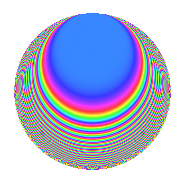Properties

 Label 3600.1.dxLevel 3600 Weight 1 Character orbit dx Rep. character $$\chi_{3600}(287,\cdot)$$ Character field $$\Q(\zeta_{20})$$ Dimension 16 Newforms 1 Sturm bound 720 Trace bound 0

Related objects

Defining parameters

 Level: $$N$$ = $$3600 = 2^{4} \cdot 3^{2} \cdot 5^{2}$$ Weight: $$k$$ = $$1$$ Character orbit: $$[\chi]$$ = 3600.dx (of order $$20$$ and degree $$8$$) Character conductor: $$\operatorname{cond}(\chi)$$ = $$300$$ Character field: $$\Q(\zeta_{20})$$ Newforms: $$1$$ Sturm bound: $$720$$ Trace bound: $$0$$

Dimensions

The following table gives the dimensions of various subspaces of $$M_{1}(3600, [\chi])$$.

Total New Old
Modular forms 256 16 240
Cusp forms 64 16 48
Eisenstein series 192 0 192

The following table gives the dimensions of subspaces with specified projective image type.

$$D_n$$ $$A_4$$ $$S_4$$ $$A_5$$
Dimension 16 0 0 0

Trace form

 $$16q$$ $$\mathstrut +\mathstrut O(q^{10})$$ $$16q$$ $$\mathstrut -\mathstrut 4q^{13}$$ $$\mathstrut +\mathstrut 4q^{37}$$ $$\mathstrut +\mathstrut 4q^{73}$$ $$\mathstrut -\mathstrut 12q^{85}$$ $$\mathstrut +\mathstrut 4q^{97}$$ $$\mathstrut +\mathstrut O(q^{100})$$

Decomposition of $$S_{1}^{\mathrm{new}}(3600, [\chi])$$ into irreducible Hecke orbits

Label Dim. $$A$$ Field Image CM RM Traces $q$-expansion
$$a_2$$ $$a_3$$ $$a_5$$ $$a_7$$
3600.1.dx.a $$16$$ $$1.797$$ $$\Q(\zeta_{40})$$ $$D_{20}$$ $$\Q(\sqrt{-1})$$ None $$0$$ $$0$$ $$0$$ $$0$$ $$q-\zeta_{40}^{7}q^{5}+(-\zeta_{40}^{4}+\zeta_{40}^{18})q^{13}+\cdots$$

Decomposition of $$S_{1}^{\mathrm{old}}(3600, [\chi])$$ into lower level spaces

$$S_{1}^{\mathrm{old}}(3600, [\chi]) \cong$$ $$S_{1}^{\mathrm{new}}(900, [\chi])$$$$^{\oplus 3}$$